Processing ......FreeComputerBooks.com Free Computer, Mathematics, Technical Books and Lecture Notes, etc.

Number Theory
Related Book Categories:
•Elementary Number Theory: Primes, Congruences, and Secrets

This is a book about prime numbers, congruences, secret messages, and elliptic curves that you can read cover to cover. Many numerical examples are given throughout the book using the Sage mathematical software.

•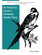An Introductory Course in Elementary Number Theory (Wissam Raji)

This book contains a useful introduction to important topics that need to be addressed in a course in number theory. Proofs of basic theorems are presented in an interesting and comprehensive way that can be read and understood even by non-majors.

•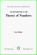An Introduction to the Theory of Numbers (Leo Moser)

This book, which presupposes familiarity only with the most elementary concepts of arithmetic (divisibility properties, greatest common divisor, etc.), is an expanded version of a series of lectures for graduate students on elementary number theory.

•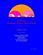Yet Another Introductory Number Theory Textbook (J. A. Poritz)

This introductory number theory textbook has a particular emphasis on connections to cryptology. It broaches the subject of these cryptologic algorithms to develop connections to the topic of number theory.

•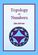Topology of Numbers (Allen Hatcher)

A textbook on elementary number theory from a geometric point of view, as opposed to the usual strictly algebraic approach. A fair amount of the book is devoted to studying Conway's topographs associated to quadratic forms in two variables.

•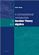A Computational Introduction to Number Theory and Algebra

This introductory book emphasises algorithms and applications, such as cryptography and error correcting codes, and is accessible to a broad audience. The coverage includes the basics of number theory, abstract algebra and discrete probability theory.

•Algorithmic Number Theory: Lattices, Curves, and Cryptography

This text provides a comprehensive introduction to algorithmic number theory for beginning graduate students, written by the leading experts in the field. It includes several articles that cover the essential topics in this area.

•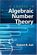A Course in Algebraic Number Theory (Robert B. Ash)

This book explores the general theory of factorization of ideals in Dedekind domains as well as the number field case. Detailed calculations illustrate the use of Kummer's theorem on lifting of prime ideals in extension fields.

•Analytic Number Theory: A Tribute to Gauss and Dirichlet

The book begins with a definitive summary of the life and work of Dirichlet and continues with thirteen papers by leading experts on research topics of current interest in number theory that were directly influenced by Gauss and Dirichlet.

•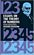Essays on the Theory of Numbers (Richard Dedekind)

This book contains the two most important essays on the logical foundations of the number system by the famous German mathematician J. W. R. Dedekind. The contents of these essays belong to the foundations of mathematics and our number system.

•A Friendly Introduction to Number Theory (Joseph H. Silverman)

This book is an introductory text designed to entice non-math majors into learning some mathematics, while at the same time teaching them how to think mathematically. The exposition is informal, with a wealth of numerical examples that are analyzed for patterns and used to make conjectures.

•Number Theory

This is the previous page of Number Theory, we are in the processing to convert all the books there to the new page. Please check this page again!!!

Book CategoriesAll CategoriesRecent BooksIT Research LibraryMiscellaneous Books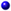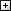Computer LanguagesComputer ScienceData Science/DatabasesElectronic EngineeringJava and Java EE (J2EE)Linux and UnixMathematicsMicrosoft and .NETMobile ComputingNetworking and CommunicationsSoftware EngineeringSpecial TopicsWeb Programming
Other Categories